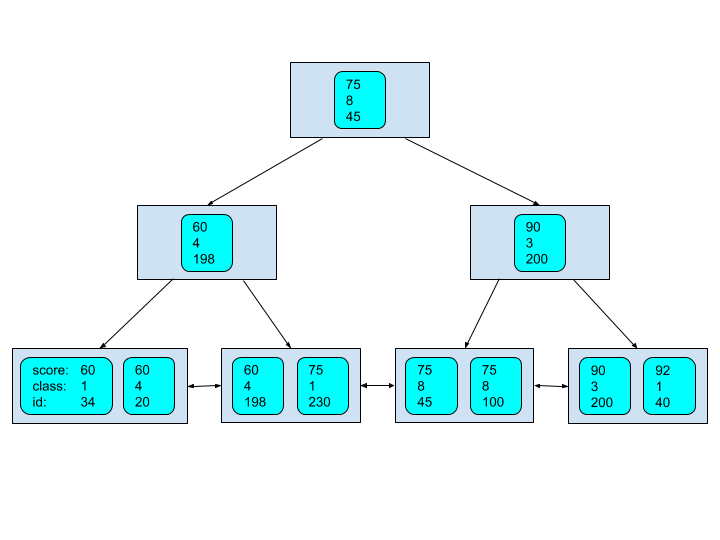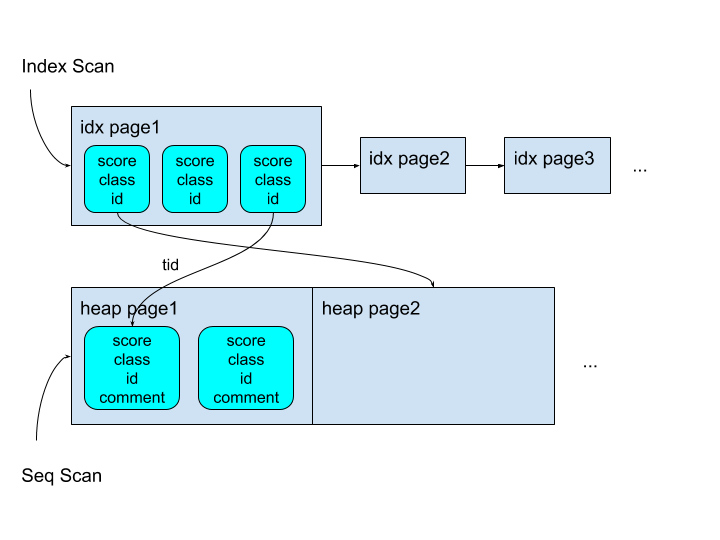# 巧用复合索引，优化查询性能

delphi6fans

0收藏

• id，学生唯一编号
• score，考试分数，0~100之间
• class，学生班级，1~10之间
• comment，学生评价，本示例中插入一个随机字符串
``````article=# create table student (id int, score int, class int, comment varchar(128)) distributed by (id);
CREATE TABLE``````

``````article=# insert into student (id, score, class, comment) SELECT g, random() * 40 + 60, random() * 10 + 1, md5(g::text) FROM generate_series(1,20000000) as g;
INSERT 0 20000000

article=# insert into student (id, score, class, comment) SELECT g, random() * 60, random() * 10 + 1, md5(g::text) FROM generate_series(20000001,20010000) as g;
INSERT 0 10000``````

``````article=# create index on student(score,class,id);
CREATE INDEX
article=# analyze student;
ANALYZE``````

``````score    class    id
60       1        34
60       4        20
60       4        198
75       1        230
75       8        45
75       8        100
90       3        200
92       1        400``````

Greenplum中其建立好的B-Tree复合索引逻辑上如下图所示，其中圆角矩形是索引元组，其中存储的键值如前文所述，按(score,class,id)排列；方角矩形是B-Tree节点/页面，每个节点内存储了多个索引元组。``````article=# show seq_page_cost;
seq_page_cost
---------------
1
(1 row)

article=# show random_page_cost;
random_page_cost
------------------
100
(1 row)

article=# set random_page_cost to 5;
SET``````

• 查询不及格的总人数
``````article=# explain (costs off) select count(*) from student where score < 60;
QUERY PLAN
-------------------------------------------------------------------------
Aggregate
->  Gather Motion 2:1  (slice1; segments: 2)
->  Aggregate
->  Bitmap Heap Scan on student
Recheck Cond: (score < 60)
->  Bitmap Index Scan on student_score_class_id_idx
Index Cond: (score < 60)
Optimizer: Postgres query optimizer
(8 rows)``````

• 查询一班中不及格的总人数
``````article=# explain (costs off) select count(*) from student where class = 1 and score < 60;
QUERY PLAN
-------------------------------------------------------------------------
Aggregate
->  Gather Motion 2:1  (slice1; segments: 2)
->  Aggregate
->  Bitmap Heap Scan on student
Recheck Cond: ((score < 60) AND (class = 1))
->  Bitmap Index Scan on student_score_class_id_idx
Index Cond: ((score < 60) AND (class = 1))
Optimizer: Postgres query optimizer
(8 rows)``````

• 查询三班的总人数
``````article=# explain (costs off) select * from student where class = 3;
QUERY PLAN
------------------------------------------
Gather Motion 2:1  (slice1; segments: 2)
->  Seq Scan on student
Filter: (class = 3)
Optimizer: Postgres query optimizer
(4 rows)``````

• 查询id=100的同学的考试信息

``````article=# explain (costs off) select * from student where id = 100;
QUERY PLAN
--------------------------------------------------------------
Gather Motion 1:1  (slice1; segments: 1)
->  Index Scan using student_score_class_id_idx on student
Index Cond: (id = 100)
Optimizer: Postgres query optimizer
(4 rows)``````

``````article=# explain analyze select * from student where id = 100;
QUERY PLAN
-------------------------------------------------------------------------------------
[...]
->  Seq Scan on student  (cost=0.00..302179.47 rows=1 width=45) (actual time=163.513..2282.622 rows=1 loops=1)
[...]
Execution time: 2304.333 ms

article=# explain analyze select * from student where id = 100;
QUERY PLAN
-------------------------------------------------------------------------------------
[...]
->  Index Scan using student_score_class_id_idx on student  (cost=0.19..245071.28 rows=1 width=45) (actual time=134.610..636.103 rows=1 loops=1)
[...]
Execution time: 637.869 ms``````• 顺序扫描SeqScan，直接读取堆中的数据元组，由于不要求元组有序，因此基本都是顺序IO，但是每个数据元组对比对应的索引元组要大一些（多了comment字段）
• 索引扫描IndexScan，

a. 需要按序读取每个页面，页面内是顺序IO，而页面之间是随机IO

b. 到heap表中读取符合索引条件的数据元组，需要大量随机IO（如果命中查询条件的元组多的话）

``````article=# select pg_relation_size('student');
pg_relation_size
------------------
1724776448
(1 row)
article=# select pg_indexes_size('student');
pg_indexes_size
-----------------
624852992
(1 row)``````